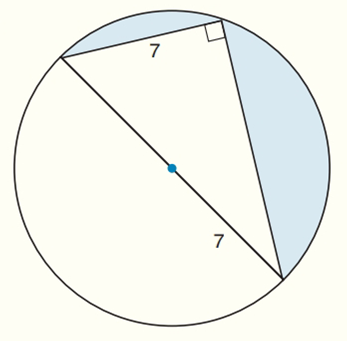Chapter 8.CR, Problem 28CRElementary Geometry For College St...

7th Edition
Alexander + 2 others
ISBN: 9781337614085

Solutions

Chapter
SectionElementary Geometry For College St...

7th Edition
Alexander + 2 others
ISBN: 9781337614085
Textbook Problem

Find the exact areas of the shaded regions in Exercises 27 to 31.To determine

To find:

The exact areas of the shaded regions.

Explanation

Calculation:

Sketch the following diagram:

The area of right angle triangle A=12bh

To find height:

The given triangle is 30-60-90-degree right triangle.

Long leg of the triangle = short leg ×3

To find short leg (a) of the triangle:

2a=142a2=142a=7

Long leg of the triangle (a3) = 73

Height (h) = 73 and base (b) = 7

To find the area of semi-circle:

Radius (r) = OC = 7

Still sussing out bartleby?

Check out a sample textbook solution.

See a sample solution

The Solution to Your Study Problems

Bartleby provides explanations to thousands of textbook problems written by our experts, many with advanced degrees!

Get Started

Evaluate the expression sin Exercises 116. (32)3

Finite Mathematics and Applied Calculus (MindTap Course List)

Use an integral to estimate the sum i=110000i.

Single Variable Calculus: Early Transcendentals, Volume I

For , f′(x) =

Study Guide for Stewart's Single Variable Calculus: Early Transcendentals, 8th

Find for defined implicity by .

Study Guide for Stewart's Multivariable Calculus, 8th

In Exercises 918, indicate whether the matrix is in row-reduced form. [0100|35]

Finite Mathematics for the Managerial, Life, and Social Sciences